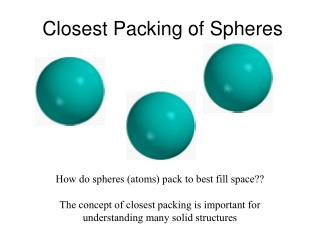DownloadDownload PresentationClosest Packing of Spheres

# Closest Packing of Spheres

Télécharger la présentation## Closest Packing of Spheres

- - - - - - - - - - - - - - - - - - - - - - - - - - - E N D - - - - - - - - - - - - - - - - - - - - - - - - - - -
##### Presentation Transcript

1. Closest Packing of Spheres How do spheres (atoms) pack to best fill space?? The concept of closest packing is important for understanding many solid structures

2. r Closest Packing of Circles Arrange circles in regular pattern with all edges touching What percentage of space do circles packed in this fashion fill? Area of rectangle = 6r x 8r = 48r2 Area filled by circles = 12 x (πr2) = 12πr2 Percentage of space filled by circles = 37.7/48 = 78.5%

3. r 4r x r base x height r2 = = 2 Area of triangle = 2 Closest Packing of Circles Arrange circles in regular pattern with all edges touching. Shift by r and nudge up to maximize packing What percentage of space does this fill? Area filled by circles = 3 x (1/6πr2) + 3 x (1/2πr2) = 2πr2 Percentage of space filled by circles = 6.28/6.93 = 91%

4. Closest Packing of Spheres a a a Imagine you are looking down at the tops of a group of spheres b b b There are two choices of hollows to place the next layer of spheres, a and b a b Note that once we choosen to put the second layer sphere into site a, we can no longer put a sphere into site b.

5. y y x x y y x x Closest Packing of Spheres Imagine you are looking down at the tops of two layers of spheres Note, we have two type of sites for the third layer, x and y. Site x is located above layer one sphere Site y is located above layer one hole Note, once again we can only fill x or y sites, not both, with new spheres

6. Closest Packing of Spheres y x Filling the x sites ABABAB…. Packing Hexagonal close packing (HCP) Filling the y sites ABCABCABC…. Packing Cubic close packing (CCP)

7. Hexagonal Close Packed Lattice

8. Cubic Close Packed Lattice

9. Coordination in HCP and CCP Each atom is surrounded by six atoms in the same plane There are an additional three atoms in the planes above and below Every atom has a total of twelve nearest neighbors

10. Let radius of spheres = r • cell diagonal = 4r • cell edge = r Volume of cube = volume of spheres = 16.76/22.63 = 0.74 volume of cube Percent of Volume Filled byClose-Packed Spheres Calculate for FCC cell: Volume of spheres = 4 x (4/3πr3) 4 atoms in cell Percent volume occupied = 74%